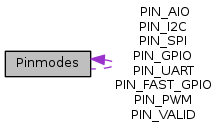mraa  2.0.0 Low Level Skeleton Library for Communication on GNU/Linux platforms
Pinmodes Class Reference
Collaboration diagram for Pinmodes:[legend]

## Public Member Functions

final int swigValue ()

String toString ()

## Static Public Member Functions

static Pinmodes swigToEnum (int swigValue)

## Static Public Attributes

static final Pinmodes PIN_VALID = new Pinmodes("PIN_VALID", mraaJNI.PIN_VALID_get())

static final Pinmodes PIN_GPIO = new Pinmodes("PIN_GPIO", mraaJNI.PIN_GPIO_get())

static final Pinmodes PIN_PWM = new Pinmodes("PIN_PWM", mraaJNI.PIN_PWM_get())

static final Pinmodes PIN_FAST_GPIO = new Pinmodes("PIN_FAST_GPIO", mraaJNI.PIN_FAST_GPIO_get())

static final Pinmodes PIN_SPI = new Pinmodes("PIN_SPI", mraaJNI.PIN_SPI_get())

static final Pinmodes PIN_I2C = new Pinmodes("PIN_I2C", mraaJNI.PIN_I2C_get())

static final Pinmodes PIN_AIO = new Pinmodes("PIN_AIO", mraaJNI.PIN_AIO_get())

static final Pinmodes PIN_UART = new Pinmodes("PIN_UART", mraaJNI.PIN_UART_get())

The documentation for this class was generated from the following file:
• Pinmodes.java